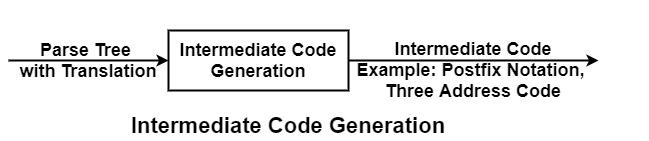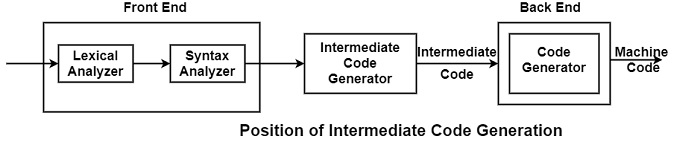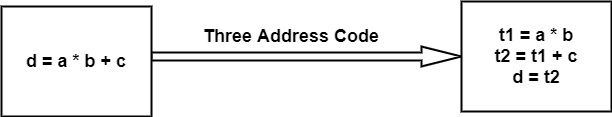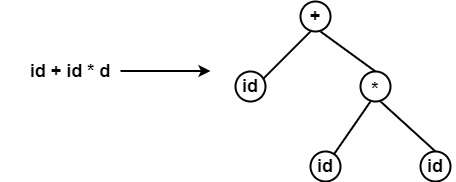# What is Intermediate Code Generation?

Intermediate code can translate the source program into the machine program. Intermediate code is generated because the compiler can’t generate machine code directly in one pass. Therefore, first, it converts the source program into intermediate code, which performs efficient generation of machine code further. The intermediate code can be represented in the form of postfix notation, syntax tree, directed acyclic graph, three address codes, Quadruples, and triples.If it can divide the compiler stages into two parts, i.e., Front end & Back end, then this phase comes in between.Example of Intermediate Code Generation −

• Three Address Code− These are statements of form c = a op b, i.e., in which there will be at most three addresses, i.e., two for operands & one for Result. Each instruction has at most one operator on the right-hand side.

Example of three address code for the statement• Postfix Notation − In postfix notation, the operator comes after an operand, i.e., the operator follows an operand.

Example

• Postfix Notation for the expression (a+b) * (c+d) is ab + cd +*

• Postfix Notation for the expression (a*b) - (c+d) is ab* + cd + - .

• Syntax Trees − It is condensed form of parse tree in which leaves are identifiers and interior node will be operators.

Example• Quadruples representation − Records with fields for the operators and operands can be used to describe three address statements. It is applicable to use a record structure with fields, first hold the operator ‘op’, next two hold operands 1 and 2 respectively, and the last one holds the result. This representation of three addresses is called a quadruple representation.
• Triples representation − The contents of operand 1, operand 2, and result fields are generally pointer to symbol records for the names defined by these fields. Therefore, it is important to introduce temporary names into the symbol table as they are generated.

This can be prevented by using the position of statement defines temporary values. If this is completed then, a record structure with three fields is enough to define the three address statements− The first holds the operator and the next two holds the values of operand 1 and operand 2 respectively. Such representation is called triple representation.

• It is Machine Independent. It can be executed on different platforms.

• It creates the function of code optimization easy. A machine-independent code optimizer can be used to intermediate code to optimize code generation.

• It can perform efficient code generation.

• From the existing front end, a new compiler for a given back end can be generated.

• Syntax-directed translation implements the intermediate code generation, thus by augmenting the parser, it can be folded into the parsing